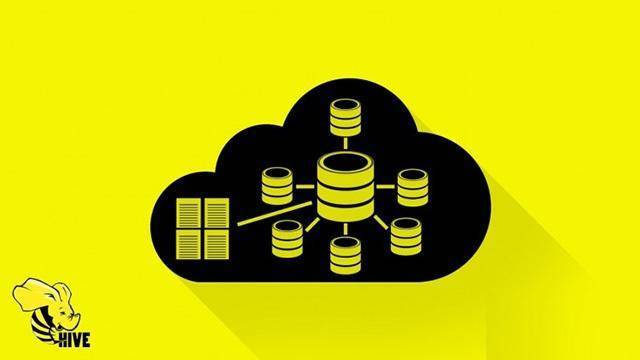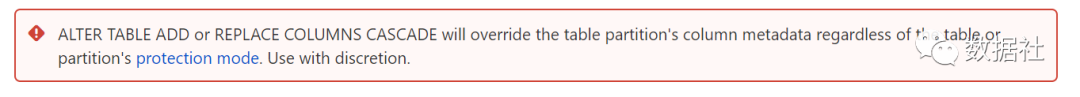# Hive 改表结构的两个坑|避坑指南Hive 在大数据中可能是数据工程师使用的最多的组件，常见的数据仓库一般都是基于 Hive 搭建的，在使用 Hive 时候，遇到了两个奇怪的现象，今天给大家聊一下，以后遇到此类问题知道如何避坑！

## 坑一：改变字段类型后更新数据不成功CREATE TABLE tb_dw_test(

insert overwrite table tb_dw_test partition (statis_date=20160501) values(1.02);

hive> select * from tb_dw_test;

ALTER TABLE tb_dw_test REPLACE COLUMNS (a DECIMAL(5,2))

insert overwrite table tb_dw_test partition (statis_date=20160501) values(1.02);

hive> select * from tb_dw_test;Determine what precision/scale you would like to set for the decimal column in the table. For each decimal column in the table, update the column definition to the desired precision/scale using the ALTER TABLE command:

ALTER TABLE foo CHANGE COLUMN dec_column_name dec_column_name DECIMAL(38,18);

If the table is a partitioned table, then find the list of partitions for the table:If the table is not a partitioned table, then you are done. If the table has partitions, then go on to step 3

SHOW PARTITIONS foo;

This can be done with a single ALTER TABLE CHANGE COLUMN by using dynamic partitioning (available for ALTER TABLE CHANGE COLUMN in Hive 0.14 or later, with HIVE-8411):Each existing partition in the table must also have its DECIMAL column changed to add the desired precision/scale.

SET hive.exec.dynamic.partition = true;

ALTER TABLE tb_dw_test PARTITION (statis_date) CHANGE COLUMN a a DECIMAL(5,2);

insert overwrite table tb_dw_test partition (statis_date=20160501) values(1.02);

hive> select * from tb_dw_test;

## 坑二：增加字段后更新数据不成功

alter table tb_dw_test add COLUMNS (b varchar(5))

hive> select * from tb_dw_test;

insert overwrite table tb_dw_test partition(statis_date=20160501) values (2.01,0371);

hive> select * from tb_dw_test;alter table tb_dw_test replace COLUMNS (a  decimal(5,2),b varchar(5)) CASCADE;

hive> select * from tb_dw_etst;

## 避坑指南## 评论